Next: Vector Calculus Up: Vectors and Vector Fields Previous: Scalar Triple Product

Vector Triple Product

For three vectors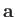,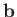, and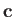, the vector triple product is defined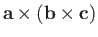. The brackets are important because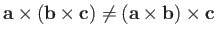. In fact, it can be demonstrated that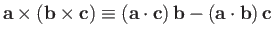(A.60)

and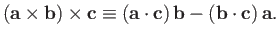(A.61)

Let us try to prove the first of the previous theorems. The left-hand side and the right-hand side are both proper vectors, so if we can prove this result in one particular coordinate system then it must be true in general. Let us take convenient axes such that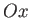lies along, andlies in the-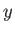plane. It follows that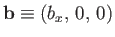,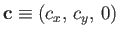, and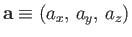. The vector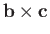is directed along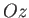: in fact,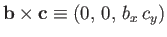. Hence,lies in the-plane: in fact,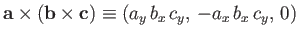. This is the left-hand side of Equation (A.60) in our convenient coordinate system. To evaluate the right-hand side, we need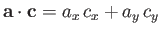and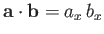. It follows that the right-hand side is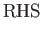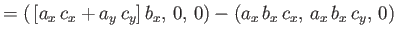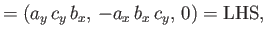(A.62)

which proves the theorem.Next: Vector Calculus Up: Vectors and Vector Fields Previous: Scalar Triple Product
Richard Fitzpatrick 2016-01-22